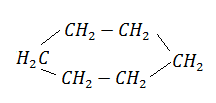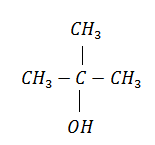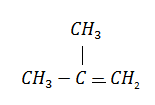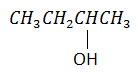Gammexane (a $\mathrm{\gamma }$-isomer) is:

1. BHC                                          2. benzene hexachloride

3. lindane                                      4. all of these

High Yielding Test Series + Question Bank - NEET 2020

Difficulty Level:

In the following reaction the catalyst used is:$\to$${\mathrm{C}}_{6}{\mathrm{H}}_{6}$ + 3${\mathrm{H}}_{2}$

1. ${\mathrm{Cr}}_{2}{\mathrm{O}}_{3}$                                      2. $A{l}_{2}{\mathrm{O}}_{3}$

3. Zn dust                                    4. ${\mathrm{Cr}}_{2}{\mathrm{O}}_{3}$ and $A{l}_{2}{\mathrm{O}}_{3}$

Concept Questions :-

Alkanes, Alkenes and Alkynes - Chemical Properties
High Yielding Test Series + Question Bank - NEET 2020

Difficulty Level:

Silver acetylide when heated with HCl gives:

(a) ${\mathrm{C}}_{2}{\mathrm{H}}_{2}$                                (b) ${\mathrm{H}}_{2}$

(c) ${\mathrm{C}}_{2}{\mathrm{H}}_{4}$                                (d) ${\mathrm{C}}_{6}{\mathrm{H}}_{6}$

High Yielding Test Series + Question Bank - NEET 2020

Difficulty Level:

$\mathrm{R}-{\mathrm{CH}}_{2}-{\mathrm{CCl}}_{2}-\mathrm{R}\stackrel{\mathrm{Reagent}}{\to }$$\mathrm{R}-\mathrm{C}\equiv \mathrm{C}-\mathrm{R}$. The reagent is

1. Na                                     2. HCl in ${\mathrm{H}}_{2}\mathrm{O}$

3. KOH in ${\mathrm{C}}_{2}{\mathrm{H}}_{5}\mathrm{OH}$                 4. Zn in alcohol

Concept Questions :-

Alkanes, Alkenes and Alkynes - Chemical Properties
High Yielding Test Series + Question Bank - NEET 2020

Difficulty Level:

Which of these will not react with acetylene?

1. NaOH                                       2. Amm. ${\mathrm{AgNO}}_{3}$

3. Na                                            4. HCl

Concept Questions :-

Alkanes, Alkenes and Alkynes - Chemical Properties
High Yielding Test Series + Question Bank - NEET 2020

Difficulty Level:

Alkene-1 on hydroboration followed with action of ${\mathrm{H}}_{2}{\mathrm{O}}_{2}$ gives:

(a) alkanol-2                                   (b) alkanol-1

(c) alkanal                                      (d) alkanone

Concept Questions :-

Alkanes, Alkenes and Alkynes - Chemical Properties
High Yielding Test Series + Question Bank - NEET 2020

Difficulty Level:

The reaction,$\stackrel{{\mathrm{H}}_{2}{\mathrm{SO}}_{4}}{\to }$is the example of:

(a) sulphonation                            (b) dehydration

(c) alkylation                                 (d) decomposition

Concept Questions :-

Alkanes, Alkenes and Alkynes - Chemical Properties
High Yielding Test Series + Question Bank - NEET 2020

Difficulty Level:

Which of the following reagents will be able to distinguish between 1-butyne and 2-butyne?

(a) ${\mathrm{NaNH}}_{2}$           (b) HCl             (c) ${\mathrm{O}}_{2}$             (d) ${\mathrm{Br}}_{2}$

Concept Questions :-

Alkanes, Alkenes and Alkynes - Chemical Properties
High Yielding Test Series + Question Bank - NEET 2020

Difficulty Level:

2-bromopentane is heated with potassium ethoxide in ethanol. The major product obtained is

1. 2-ethoxypentane               2. pentene-1

3. trans-pentene-2                4. cis-pentene-2

Concept Questions :-

Alkanes, Alkenes and Alkynes - Chemical Properties
High Yielding Test Series + Question Bank - NEET 2020

Difficulty Level:

An alkene, obtained by the dehydration of an alcohol (A), on ozonolysis gives two molecules of acetaldehyde for every molecule of alkene. The alcohol (A) is:

(a) ${\mathrm{CH}}_{3}{\mathrm{CH}}_{2}{\mathrm{CH}}_{2}\mathrm{OH}$                             (b) ${\mathrm{CH}}_{3}{\mathrm{CH}}_{2}\mathrm{OH}$

(c) ${\mathrm{CH}}_{3}\mathrm{CH}={\mathrm{CHCH}}_{2}\mathrm{OH}$                       (d)Concept Questions :-

Alkanes, Alkenes and Alkynes - Chemical Properties